# Caleb, Inc. determined the total cost to manufacture 25 units of Job SS5 was \$1,025. To...

Caleb, Inc. determined the total cost to manufacture 25 units of Job SS5 was \$1,025. To determine a selling price for its jobs, Moody marks up total manufacturing costs by an additional 80%. What is the per unit selling price of Job SS5?

 a. \$73.80 b. \$90.20 c. \$32.80 d. \$41.80

 Total cost = \$1025 Sale revenue = \$1025*1.80 =\$1845 Selling price per unit = total revenue / number of units =\$1845/25 =\$73.8 Correct Option : a.\$73.8
##### Add Answer of: Caleb, Inc. determined the total cost to manufacture 25 units of Job SS5 was \$1,025. To...
Similar Homework Help Questions
• ### 12. If Job P included 20 units, what was its unit product cost? 13. If Job...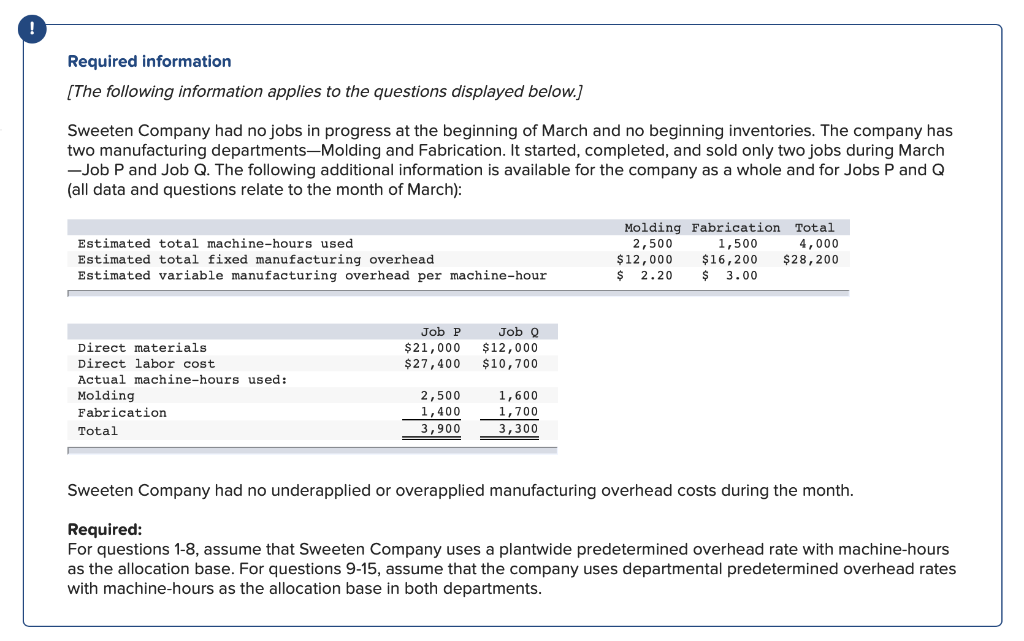12. If Job P included 20 units, what was its unit product cost? 13. If Job Q included 30 units, what was its unit product cost? 14. Assume that Sweeten Company used cost-plus pricing (and a markup percentage of 80% of total manufacturing cost) to establish selling prices for all of its jobs. What selling price would the company have established for Jobs P and Q? What are the selling prices for both jobs when stated on a per unit...

• ### Simple Metal​ Works, Inc. will manufacture and sell 200,000 units next year. Fixed costs will total...

Simple Metal​ Works, Inc. will manufacture and sell 200,000 units next year. Fixed costs will total \$330,000​,and variable costs will be 50 percent of sales. a. The firm wants to achieve a level of earnings before interest and taxes of \$250,000. What selling price per unit is necessary to achieve this​ result? b. Set up an analytical income statement to verify your solution to part (a​).

• ### 1. If Job Q included 30 units, what was its unit product cost? 2. Assume that...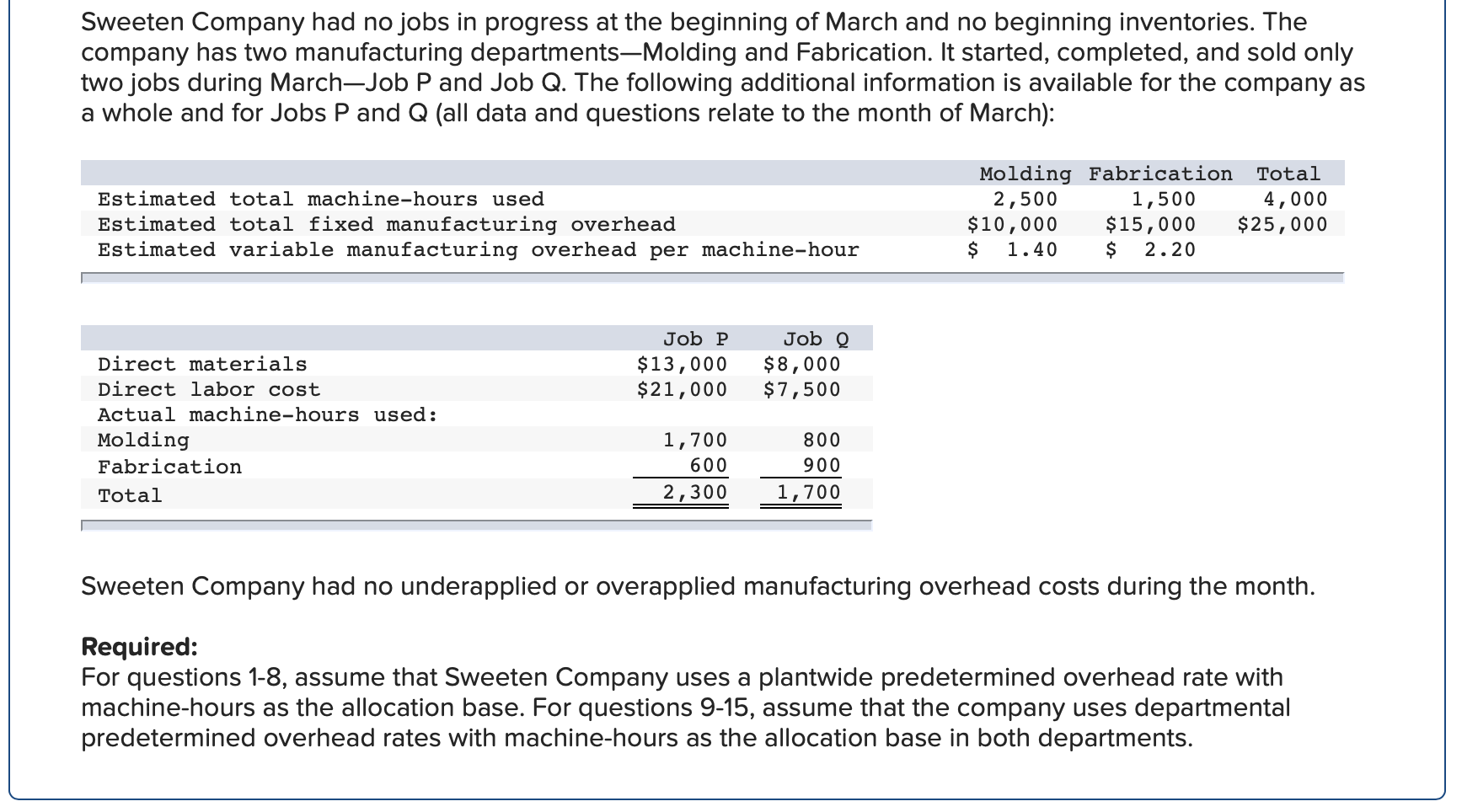1. If Job Q included 30 units, what was its unit product cost? 2. Assume that Sweeten Company used cost-plus pricing (and a markup percentage of 80% of total manufacturing cost) to establish selling prices for all of its jobs. What selling price would the company have established for Jobs P and Q? What are the selling prices for both jobs when stated on a per unit basis assuming 20 units were produced for Job P and 30 units were...

• ### 6. If Job Q included 30 units, what was its unit product cost? (Do not round...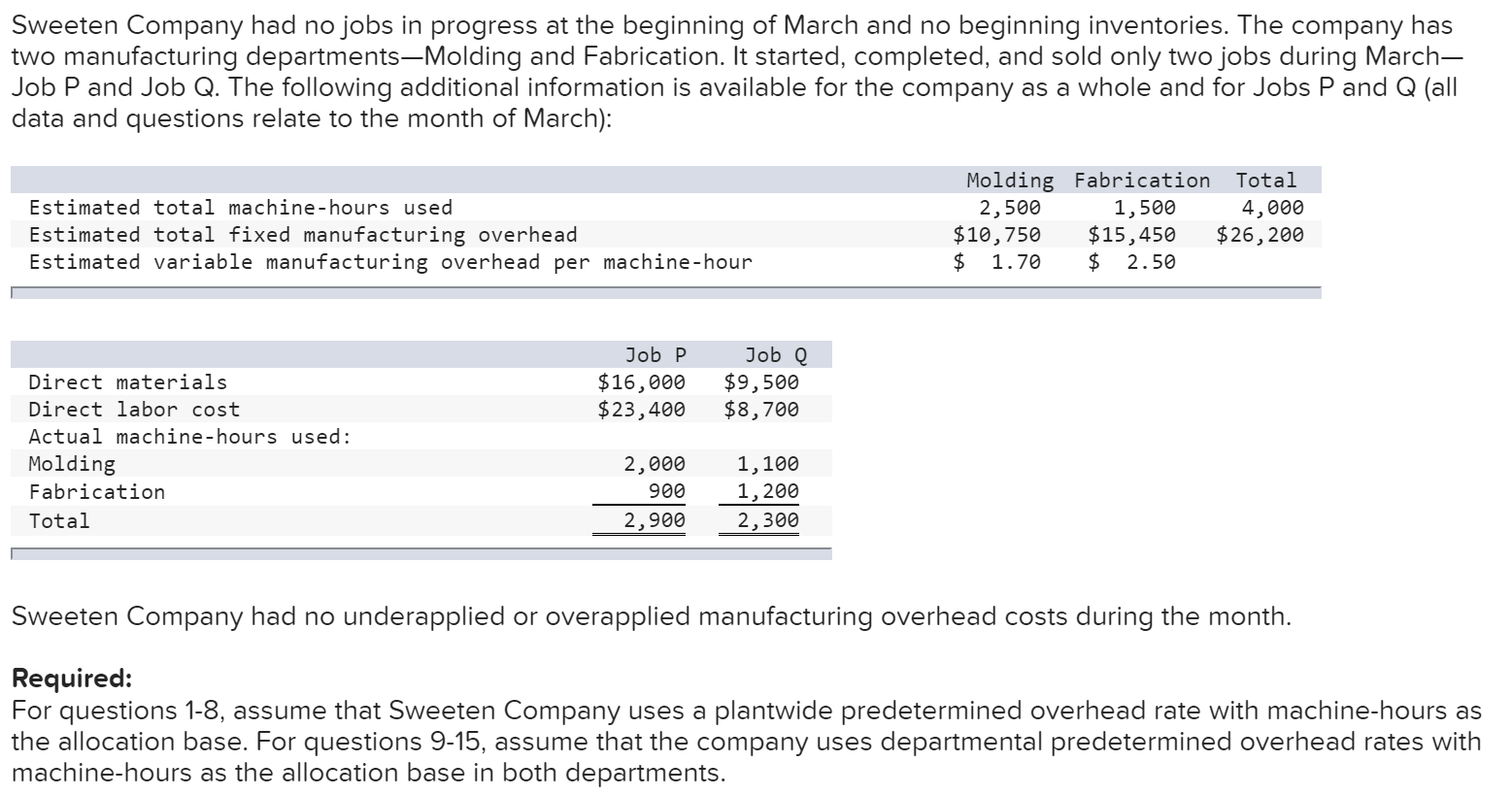6. If Job Q included 30 units, what was its unit product cost? (Do not round intermediate calculations. Round your final answer to nearest whole dollar.) 7. Assume that Sweeten Company used cost-plus pricing (and a markup percentage of 80% of total manufacturing cost) to establish selling prices for all of its jobs. What selling price would the company have established for Jobs P and Q? What are the selling prices for both jobs when stated on a per unit...

• ### 1. If Job P included 20 units, what was its unit product cost? 2. What was...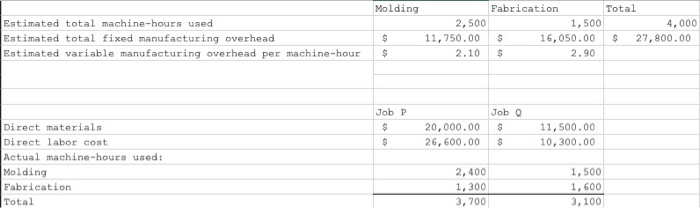1. If Job P included 20 units, what was its unit product cost? 2. What was the total manufacturing cost assigned to Job Q? 3. If Job Q included 30 units, what was its unit product cost? 4. Assume that Sweeten Company used cost-plus pricing (and a markup percentage of 80% of total manufacturing cost) to establish selling prices for all of its jobs. What selling price would the company have established for Jobs P and Q? What are the...

• ### Job P = \$ 68,145 Job Q = predetermined overhead rate * Actual Total machine hours...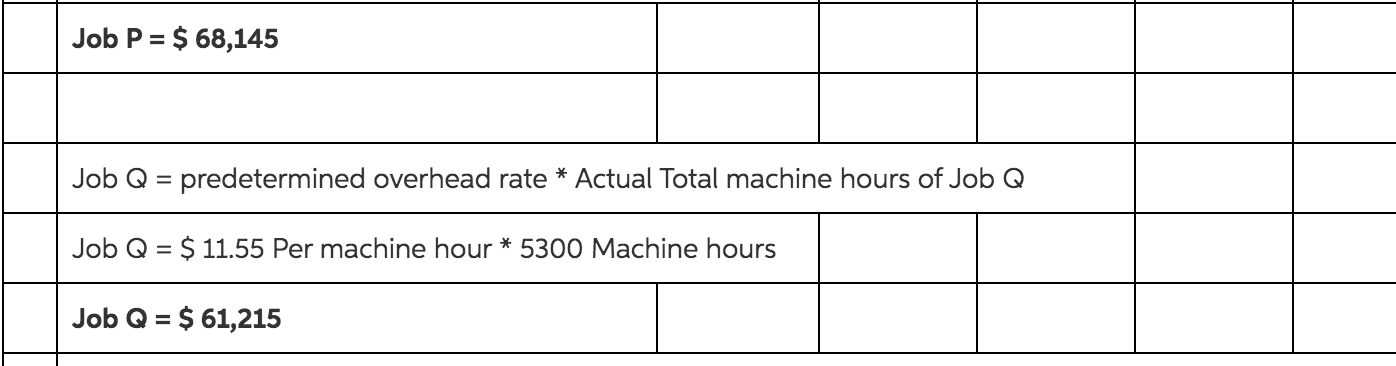Job P = \$ 68,145 Job Q = predetermined overhead rate * Actual Total machine hours of Job Q Job Q = \$ 11.55 Per machine hour * 5300 Machine hours Job Q = \$ 61,215 [The following information applies to the questions displayed below.] Sweeten Company had no jobs in progress at the beginning of March and no beginning inventories. The company has two manufacturing departments--Molding and Fabrication. It started, completed, and sold only two jobs during March-Job P...

• ### ​(​Break-even point and selling price​) Specialty​ Steel, Inc. will manufacture and sell 190,000 units next year....

​(​Break-even point and selling price​) Specialty​ Steel, Inc. will manufacture and sell 190,000 units next year. Fixed costs will total ​\$330,000​, and variable costs will be 50 percent of sales. a. The firm wants to achieve a level of earnings before interest and taxes of ​\$260,000. What selling price per unit is necessary to achieve this​ result? b. Set up a pro forma income statement to verify your solution to part a

• ### 13. If Job Q included 30 units, what was its unit product cost? 14. Assume that...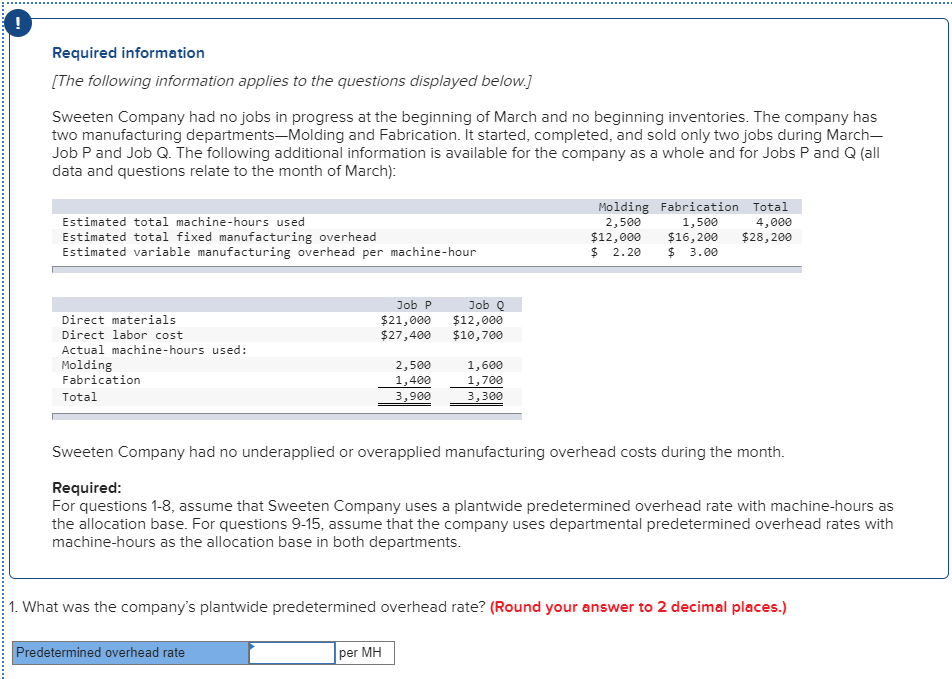13. If Job Q included 30 units, what was its unit product cost? 14. Assume that Sweeten Company used cost-plus pricing (and a markup percentage of 80% of total manufacturing cost) to establish selling prices for all of its jobs. What selling price would the company have established for Jobs P and Q? What are the selling prices for both jobs when stated on a per unit basis assuming 20 units were produced for Job P and 30 units were...

• ### 13. If Job Q included 30 units, what was its unit product cost? (Do not round...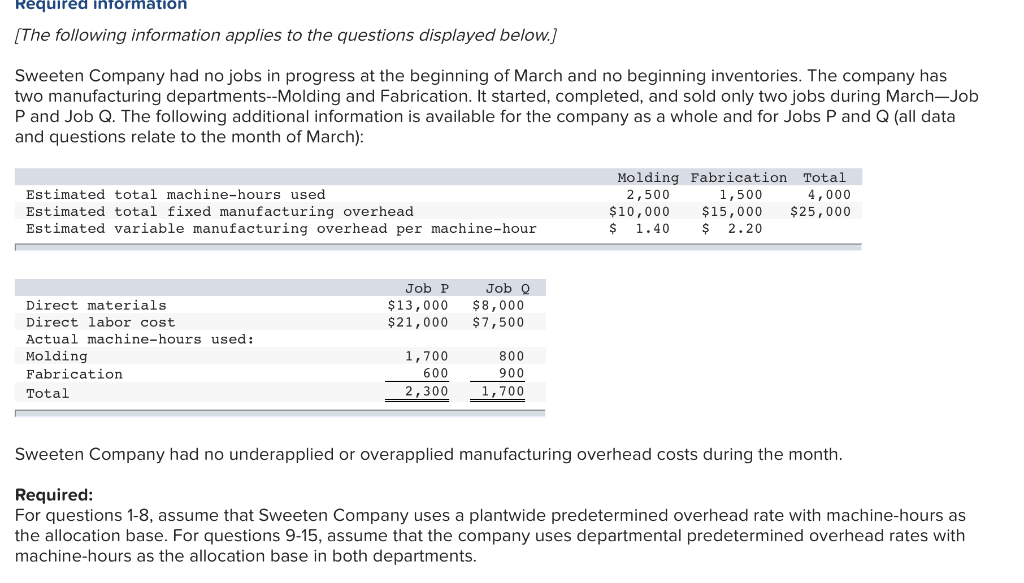13. If Job Q included 30 units, what was its unit product cost? (Do not round intermediate calculations. Round your final answer to nearest whole dollar.) 14. Assume that Sweeten Company used cost-plus pricing (and a markup percentage of 80% of total manufacturing cost) to establish selling prices for all of its jobs. What selling price would the company have established for Jobs P and Q? What are the selling prices for both jobs when stated on a per unit...

• ### 13. If Job Q included 30 units, what was its unit product cost? (Do not round...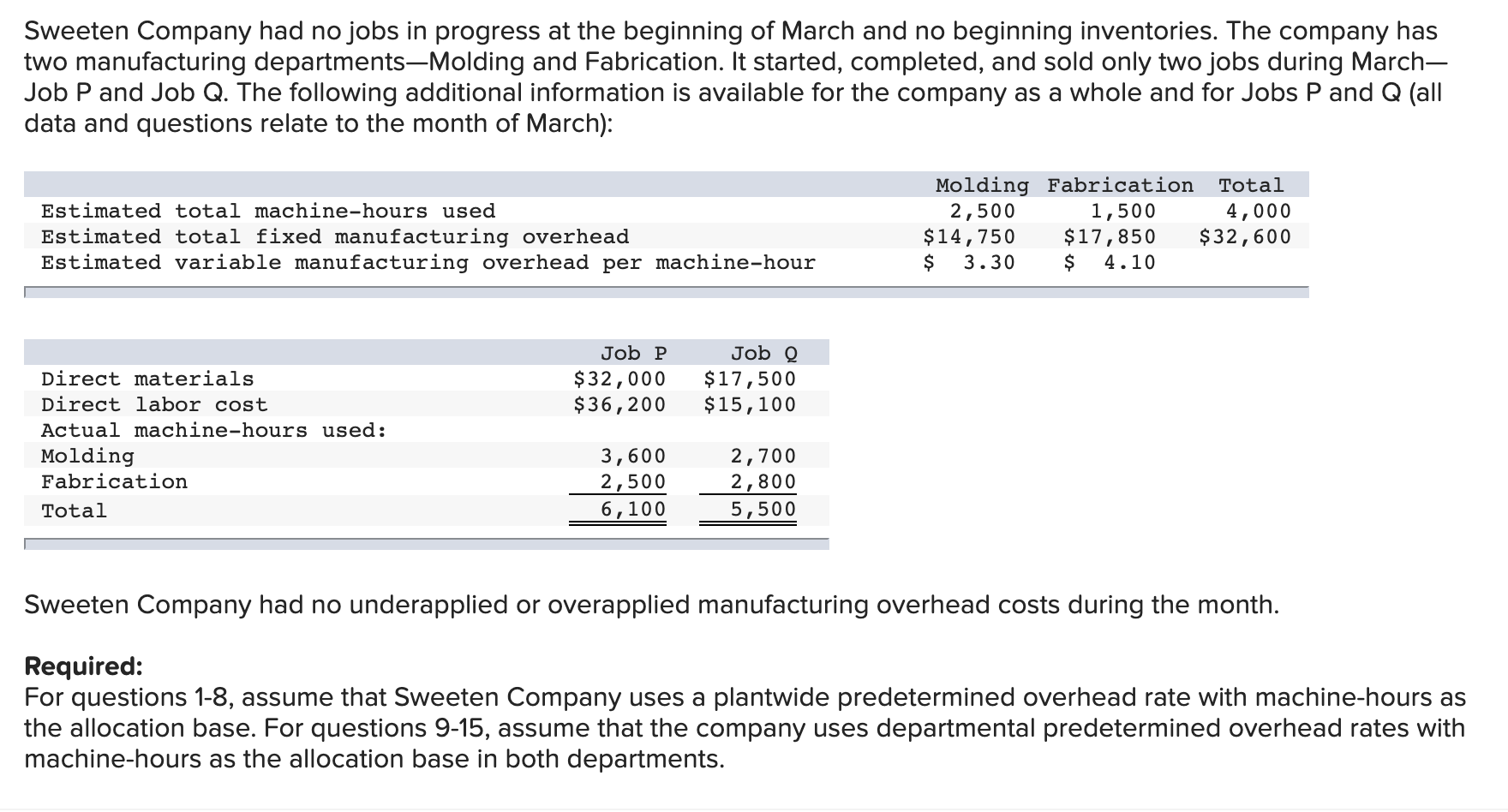13. If Job Q included 30 units, what was its unit product cost? (Do not round intermediate calculations. Round your final answer to nearest whole dollar.) 14. Assume that Sweeten Company used cost-plus pricing (and a markup percentage of 80% of total manufacturing cost) to establish selling prices for all of its jobs. What selling price would the company have established for Jobs P and Q? What are the selling prices for both jobs when stated on a per unit...

Free Homework App